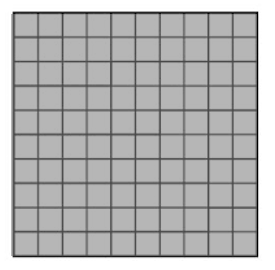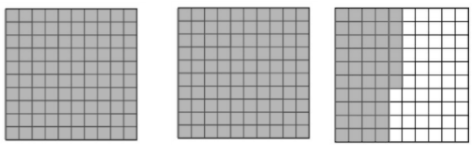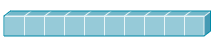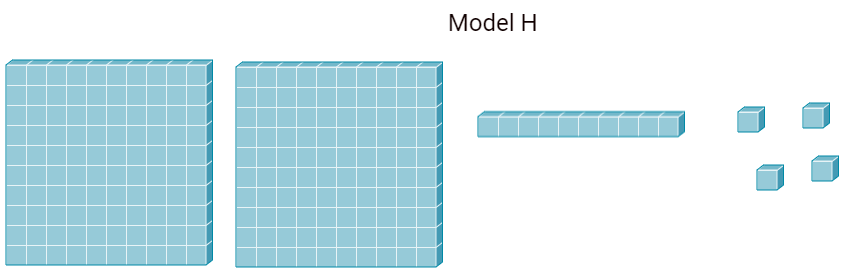SOL 4.3 abc
SOL 4.4d (Desmos Calculator allowed)
SOL 4.5a (Desmos Calculator allowed)
SOL 4.6b (Desmos Calculator allowed)
SOL 4.16 (Desmos Calculator allowed)
100
1. This figure is shaded to represent the number 1What is the number shown below?A) 246      B) 20.46       C) 2.46         D) 2.046

C) 2.46

100

A farmer planted 1,253 pumpkin plants in a field last year. This year the farmer planted 3,186 pumpkin plants. How many more pumpkin plants did the farmer plant this year than last year?

The farmer planted 1,933 more plants this year than last.

3,186 - 1,253 = 1,933

100

What numbers are common factors of 8 and 36?

1      2      3      4      6       8      12    18    36

1     2      4     are common factors. Common factors must divide evenly into both numbers.

100
1. Yolanda bought a cheeseburger for \$6.85 and some onion rings for \$1.79, including tax. She gave the cashier \$10 to pay for these items. What is the amount of change Yolanda should receive?

A )  \$8.64      B)  \$1.36     C)  \$18.64     D)  \$1.46

Yolanda's change would be \$1.36

Yolanda's total is \$8.64 for her cheeseburger and onion rings. If she pays with \$10, I subtract the food total from \$10 to find what her change would be.

100
1. Which statement is true?

A)    6 × 4 = 24 + 24

B)   7 × 8 = 80 - 19

C)   9 × 6 = 79 - 25

D)   6 × 7 = 40 + 12

The true statement would be C) 9 x6 = 79-25

9 x 6 is 54, and 79-20 is 59, then subtract 5 more is 54.

I knew A was not an option since 6 x4 is 24, and 24 + 24 would be bigger than just 24.

40 + 10 is 50, and then add 2 more would be 52, and 7 x 6 is 42, so D couldn't be correct.

80 -20 is 60, then I add 1 back in since it is 80-19, so it's 61. 7 x 8 is 48, so B can't be the answer.

200
1. This model represents one whole.Which decimal is represented by Model H?

A) 214              C)  2.14

B)  21.4            D)  0.214B)  21.4

200

Mrs. Vaughan’s class has a goal of selling 500 cookies in three months.  The class sold 95 cookies the first month  The class sold 276 cookies the second month Exactly how many more cookies does the class need to sell during the third month to reach its goal?

The class needs to sell 129 more cookies to meet its goal.

If I start with the goal of 500, and I subtract from the goal month 1 (95) and month 2 ( 276), then they still need 129 more to reach 500.

200
1. What is the least common multiple of 6, 8, and 12?

A   2

B   12

C   24

D   48

The LCM, or least common multiple of 6, 8, and 12 is 2.

12 isn't a multiple of 8, and while 24 and 48 are common multiples, they aren't the smallest ones that 6, 8, and 12 share.

200
1. The table shows the weight of fish caught at a fishing tournament.

Place in Tournament

Total Weight of Fish (pounds)

1st

12.78 lbs.

2nd

11.97 lbs.

3rd

11.52  lbs.

What is the difference, in pounds, between 1st and 2nd place?

The difference in pounds ( lbs) between 1st and 2nd place is 0.81 pounds (lbs)

I have to subtract to find my answer, and I have to have my bigger number on top, so I subtract second place from first place.

200

Write the correct symbol to make the statement correct. (= or ≠)

36 ÷ 4 ____  3 × 3

These are equal.

36 divided by 4 is 9, and 3 x 3 is also 9

300

Round 67.612 to the nearest whole number.

68

300

There are 14 tennis teams competing in a tournament. Each team has 6 tennis players. How many tennis players are there altogether?

There are 84 tennis players altogether.

If I take the 14 teams, and multiply by 6 since there are six players on each team, then there are 84 players.

300

What is the LCM of 6 and 7?

The LCM would be 42, since that is the first multiple shared in common between 6 and 7.

300

Janiya earned 17.2 points for a book she read. She passed another quiz that earned her 43.5 points. How many points does Janiya have now?

Janiya has 60.7 points in all, since I need to add the two totals.

300

Write the correct symbol to make the statement correct. (= or ≠)

2 × 10 ____ 20 - 5

They are NOT equal.

2 x 10 is 20, and 20-5 is 15

400
1. Order the numbers from least to greatest.

1.01            0.425            0.5               0.22

0.22

0.425

0.5

1.01

400

Each floor of an office building has exactly 72 workers. What is the total number of workers in the 36-floor building?

There are 2,592 workers in the building.

If each floor has 72 workers, and there are 36 floors in the building, I need to multiply these two together to find how many in all.

400

What is the GCF of  4 and 12?

The GCF of 4 and 12 would be 4.

The factors of 4 are: 1, 2, and 4

The factors of 12 are: 1, 2, 3, 4, 6, and 12

400

Mark helps out at his mother’s office, and she is weighing packages for shipping. She asked Mark to record the weights for her and let her know the total, so she can figure the shipping cost. The packages weigh 1.056 pounds, 3.158 pounds, and 2.504 pounds. What is the total weight of all the packages?

All three packages together weigh 6.718 pounds.

400

Write the correct symbol to make the statement correct. (= or ≠)

47 - 15 ____ 4 × 8

They are equal.

47- 15 is 32, and 4 x 8 is also 32

500
1. Which number is less than 7.04?

A)  7.11              B)   7.52               C )   7.008

D)  8.01

C) 7.008

500

There are 32 students in a fourth-grade classroom. Each table in the classroom seats six students. How many tables does the teacher need in the classroom to seat everyone?

The teacher will need 6 tables. Each table seats six students. When I divide 32 by 6, I get 5, remainder 2. The remainder of two stands for two students since my dividend really is students ( the 32). So since the remaining two students need a seat as well, I must add another table to my quotient of 5.

500

The GCF of 8 and 17 would be?

The GCF of 8 and 17 would be 1, since 17 is a prime number and the only factors are 1 and 17.

500

Bella had some ribbon to make a wreath. She knew she needed a total of 5.1 yards of ribbon to make the wreath. She had to buy 3.9 yards. How many yards did Bella have before she bought any ribbon?

Bella had 1.2 yards of ribbon to start, and I found this answer by subtracting what she bought ( 3.9 yds) from what she needed ( 5.1 yds)

500

What number needs to go in the blank to make this equation true?

56 ÷ 8 = 7 x ____

1 would need to go in the blank,since 56 divided by 8 is 7, and 7 x 1 =7

Click to zoom